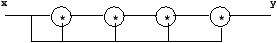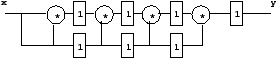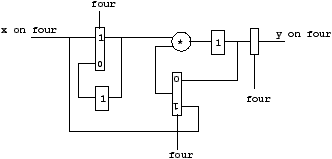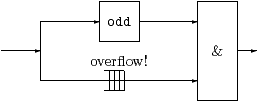## 1.2  Multi-clock systems

Up to now, we have only considered length preserving functions, that is, functions returning n items of their output when receiving n items of their input. We consider now a more general case allowing to sample stream and to compose them. This is achieved through the use of clocks.

### 1.2.1  Sampling: the operator when

when is a sampler that allows fast processes to communicate with slower ones by extracting sub-streams from streams according to a condition, i.e. a boolean stream.

 true t t t t … c f t f t … x x0 x1 x2 x3 … x when c x1 x3 … true on c f t f t …

The sampling operators introduce the notion of clock type. These clock types give some information about the time behavior of stream programs.

The clock of a stream s is a boolean sequence giving the instants where s is defined. Among these clocks, the base clock stands for the constant stream `true`: a stream on the base clock is present at every instant. In the above example, the current value of `x when c` is present when `x` and `c` are present and `c` is true. Since `x` and `c` are on the base clock `true`, the clock of `x when c` is noted `true on c`.

Consider the `sum` function which make the sum of its input (that is, sn = Σi = 0n xi).

```let node sum x = s where rec s = x -> pre s + x
```

Now the `sum` function can be used at a slower rate by sampling its input stream:

```let node sampled_sum x y = sum(x when y)

val sampled_sum :  int -> bool => int
val sampled_sum :: ’a -> (_c0:’a) -> ’a on _c0
```

`sampled_sum` has a function clock which follows its type structure. This clock type says that for any clock `'a`, if the first argument of the function has clock `'a` and the second argument named `_c0` has clock `a`, then the result is on clock `'a on _c0` (every expression variable is renamed in the clock to avoid name conflicts). An expression on clock `'a on _c0` is present when the clock `'a` is true and the boolean stream `_c0` is present and true.

Now, the sampled sum can be instantiated with an actual clock. For example:

```(* a counter that counts modulo n *)
let node sample n =
let rec cpt = 0 -> if pre cpt = n - 1 then 0 else pre cpt + 1
and ok = cpt = 0 in
ok

(* defining a 1/10 clock *)
let clock ten = sample 10

(* sampling a sum on 1/10 *)
let node sum_ten dx = sampled_sum dx ten

val ten :  bool
val ten_hz :: ’a
val sum_ten :  int => int
val sum_ten :: ’a -> ’a on ten
```

A clock name is introduced with the special keyword `clock` which builds a clock from a boolean stream.

Warning: Clocks provide a way to define control structures, that is, pieces of code which are executed according to some condition. It is thus important to understand their combination with delay operators as exemplified in the diagram below:

 c f t f t f … 1 1 1 1 1 1 … sum 1 1 2 3 4 5 … (sum 1) when c 2 4 … 1 when c 1 1 … sum (1 when c) 1 2 … x x0 x1 x2 x3 x4 … x when c x1 x3 … pre x nil x0 x1 x2 x3 … pre (x when c) nil x1 … (pre x) when c x0 x2 …

As soon as a function f contains some delay operator, sampling its inputs is not equivalent to sampling its outputs, that is, f(x when c) ≠ (f xwhen c.

Clocks can be arbitrarily nested. Consider, for example, the description of a (real) clock.

```let clock sixty = sample 60
let node hour_minute_second second =
let minute = second when sixty in
let hour = minute when sixty in
hour,minute,second

val sixty :  bool
val sixty :: ’a
val hour_minute_second :  ’a => ’a * ’a * ’a
val hour_minute_second :: ’a -> ’a on sixty on sixty * ’a on sixty * ’a
```

A stream on clock `'a on sixty on sixty` is only present one instant over 3600 instants which match perfectly what we are expecting.

Warning: We make a special treatment for clocks defined at top-level (as the clock `sixty`). A top-level clock is defined by a boolean expression (combinatorial or sequential) and is then considered as a constant process which can be instanciated several times. In the above program, they are two instanciations of the clock `sixty`: one is on some clock `'a` whereas the other run slowly at clock `'a on sixty`.

### 1.2.2  Combining Sampled Streams: the operator merge

merge conversely allows slow processes to communicate with faster ones by merging sub-streams into “larger” ones:

 c f t f f f t … x x0 x1 … y y0 y1 y2 y3 … merge c x y y0 x0 y1 y2 y3 x1 …

For instance, the `merge` allows us to define an “holding” function (the “current” operator of Lustre), which “holds” a signal between two successive samples (here `ydef` is a default value used before any sample has been taken):

```let node hold ydef c x = y
where rec y = merge c x ((ydef -> pre y) whenot c)

val hold :  ’a -> bool -> ’a => ’a
val hold :: ’a -> (_c0:’a) -> ’a on _c0 -> ’a
```

 c f t f f f t … x x0 x1 … y y0 y1 y2 y3 … ydef d0 d1 d2 d3 d4 d5 … hold c x ydef d0 x0 x0 x0 x0 x1 …

Warning: Note the difference between `merge` and `if/then/else`. `merge` composes two complementary sequences and thus, has a lazy flavor. It is the data-flow version of the classical (lazy) conditional of sequential programming languages. The `if/then/else` is a strict operator which needs its three arguments to be on the same clock 2.

The following operator is a classical control engineering operator, available in both the Scade/Lustre library and “digital” library of Simulink. Its graphical representation in Scade/Lustre is given in figure 1.2. This operator detects a rising edge (false to true transition). The output becomes true as soon as a transition has been detected and remains unchanged during `number_of_cycle` cycles. The output is initially false and a rising edge occurring while the output is true is detected. In Lucid Synchrone syntax, this is written 3:Figure 1.2: A classical operator in Scade/Lustre

```let node count_down (res, n) = cpt where
rec cpt = if res then n else (n -> pre (cpt - 1))

let node rising_edge_retrigger rer_input number_of_cycle = rer_output where
rec rer_output = (0 < v) & clk
and v = merge clk (count_down ((count,number_of_cycle) when clk))
((0 fby v) whenot clk)
and c = false fby rer_output
and clock clk = c or count
and count = false -> (rer_input & pre (not rer_input))
```

### 1.2.3  Oversampling

Using clocks defined globally, we can write simple over-sampling functions, i.e. functions whose output has a larger rate than the input. One simple example is the stuttering function which computes the sequence (on)nIN such that:

 o2n = xn o2n+1 = xn

It is written as follows:

```let clock half = h where rec h = true -> not (pre h)
let node over x = hold 1 half x
let node stuttering () =
let rec nat = 0 -> pre nat + 1 in
over nat

val half :  bool
val half :: ’a
val over :  int => int
val over :: ’a on half -> ’a
val stuttering :  unit => int
val stuttering :: ’a -> ’b
```

This is an example of oversampling, that is, a function whose internal clock (here `'a`) is faster than the clock of its input (here `'a on half`): the function `stuttering` computes some internal value whereas it does not receive any new input. It shows that some limited form of oversampling — which is possible in Signal and not in Lustre — can be achieved.Figure 1.3: Duplication vs iteration for the computation of x5

Oversampling appear naturally in a system when considering program transformation and refinements. For example, when the architecture does not offer enough parallelism, we replace it by iteration and this has some consequence on the instant where the results become available. Consider, for example, the computation of the power of a sequence (yn)nIN such that:

 yn = (xn)5

Supposing that the machine is able to execute four multiplications, we simply write:

```let node spower x = y where y = x * x * x * x * x
let node shift_power x = y where y = 1 fby (x * x * x * x * x)

val spower :  int => int
val spower :: ’a -> ’a
val shift_power :  int => int
val shift_power :: ’a -> ’a
```

The graphical representation is given on the left of figure 1.3. The output is available at the same (logical) instant as the input is received and this is why the function gets clock `'a -> 'a`.

`shift_power` is another version obtained by inserting a delay at the end of the computation. Now, a pipelined version can be obtained by a simple retiming transformation, leading to a speed-up of four in average.

```let node ppower x = y where
rec x2 = 1 fby x * x
and px = 1 fby x
and x3 = 1 fby x2 * px
and ppx = 1 fby px
and x4 = 1 fby x3 * ppx
and pppx = 1 fby ppx
and y = 1 fby x4 * pppx

val ppower :  int => int
val ppower :: ’a -> ’a
```
 x x0 x1 x2 x3 x4 x5 x6 ... spower x x05 x15 x25 x35 x45 x56 x65 ... shift_power x 1 x05 x15 x25 x35 x45 x56 ... ppower x 1 1 1 1 x05 x15 x25 ...

Now, suppose that the machine has only one multiplier instead of four. Then, the value xn5 cannot be obtained in one cycle. We replace parallel computation by iteration, making the clock of `x` five times slower. The new system is given on the right of figure 1.3.

```let clock four = sample 4

let node tpower x = y where
rec i = merge four x ((1 fby i) whenot four)
and o = 1 fby (i * merge four x (o whenot four))
and y = o when four

val tpower :  int => int
val tpower :: ’a on four -> ’a on four
```
 five t f f f t f f f t f f f t f ... x x0 x1 x2 x3 ... i x0 x0 x0 x0 x1 x1 x1 x1 x2 x2 x2 x2 x3 x3 ... o 1 x02 x03 x04 x05 x12 x13 x14 x15 x22 x23 x24 x25 x32 ... tpower x 1 x05 x15 x25 ...

`spower x` is a time refinement of the computation of `shift_power`, it produces the same sequence of values. We have made the internal clock faster than the input/output clock in order to exhibit every step of the computation.

As a consequence of the clock discipline and clock inference, using `tpower` in place of `shift_power` will automatically slowdown the whole system. This guaranty modular design, that is, `tpower` can be used anywhere `power` was previously used.

Remark: The current version of the compiler does not take the value of clocks into account (that is, it is unable to know that `four` is a periodic clock). such that it can ensure the equivalence between the various designs.x x0 x1 x2 x3 x4 x5 x6 ... half t f t f t f t ... x when half x0 x2 x4 x6 ... x & (x when half) x0  &  x0 x1  &  x2 x2  &  x4 x3  &  x6 ...
 Figure 1.4: A non Synchronous Example

### 1.2.4  Clock constraints and error messages

Program must follow some clocking rules. For example, the and gate `(&)` expects its two arguments to be booleans and to be on the same clock, as expressed by the following signatures:

```val (&) : bool -> bool -> bool
val (&) :: ’a -> ’a -> ’a
```

Thus, writting the following program leads to a clocking error:

```let clock half = sample 2
let node odd x = x when half
let node wrong_clocked x = x & odd x
```

where `wrong_clocked x` combines the stream `x` to the sub-stream of `x` made by filtering one item over two. Thus it should compute the sequence (xn & x2n)nIN. Note that this computation is clearly not bounded memory through it is only composed of bounded memory operators. The corresponding Kahn network  of `wrong_clocked x` is depicted in figure 1.4. Indeed `&` consumes one item of its two inputs in order to produce one item of its output. One of its two inputs is twice faster than the second one and, as time goes on, the number of successive values to store in a buffer will increase and the system will eventually stop. Whereas the sequence (xn & x2n)nIN is perfectly well defined, it will be considered as a non synchronous computation — its corresponding Kahn Network cannot be executed without buffering — and shall be statically rejected by the compiler. When given to the compiler, we get:

```File "tutorial.ls", line 78, characters 31-36:
>let node wrong_clocked x = x & odd x
>                               ^^^^^
This expression has clock ’a on half,
but is used with clock ’a.
```

`x` gets clock `'b` whereas `odd x` gets clock `'b on half` and these two clocks cannot made be equal. Clock constraints in Lucid Synchrone insure that the corresponding Kahn network can be executed without synchronization mechanisms using possibly unbounded buffering. This is why we reject it when dealing with real-time applications.

### 1.2.5  Equality and scope restrictions in the use of clocks

When a clock name is introduced with the `let clock` constructor, the name is considered to be unique and does not take into account the expression on the right-hand side of the `let clock`. Thus, the following program is rejected:

```let node wrong () =
let clock c = true in
let v = 1 when c in
let clock c = true in
let w = 1 when c in
v + w
```

and we get the following error:

```File "tutorial.ls", line 62, characters 6-7:
>  v + w
>      ^
This expression has clock ’b on ?_c1,
but is used with clock ’b on ?_c2.
```

Because of the inference aspect of the clock calculus, some restriction are imposed on the use of clocks 4. The main restriction is that it is not possible to return a value which depends on some clock computed locally 5.

When clocks are introduced with the `clock` constructions, these clock must not escape their lexical scope. For example:

```let node within min max x = o where
rec clock c = (min <= x) & (x <= max)
and o = true when c

File "tutorial.ls", line 123-125, characters 4-75:
>....node within min max x = o where
> rec clock c = (min <= x) & (x <= max)
> and o = true when c
The clock of this expression, that is, ’b -> ’b on ?_c0
depends on ?_c0 which escape its scope.
```

This program is rejected because the `let clock` construction introduces a fresh clock name `?_c0` which abstract the exact value of `c`. This name must not exist already, that is, it must not appear in the clock of some existing variable when clocking the `let/clock` construct and it cannot escape its local scope. Here, the program is rejected since the function returns an expression on clock `'a on ?_c0` but `?_c0` must stay local to its block structure.

```let node escape x =
let clock c = true in
merge c (x + (1 when c)) 0

File "tutorial.ls", line 123-125, characters 4-75:
>....node escape x =
>  let clock c = true in
>  merge c (x + (1 when c)) 0
The clock of this expression, that is, ’b on ?_c0 -> ’b
depends on ?_c0 which escape its scope.
```

The program is rejected because the variable `x` should already be on the clock `'a on ?_c0` in which `?_c0` appears. This fresh name must not escape the scope of the construction.

Remark: Thus, clocks introduced by the `clock` construction have a lifetime limited to their block. This is an important restriction of Lucid Synchrone V3 whose clock calculus is strictly less expressive than the one of V1. In this version (as well as in Lustre), a function may return a value sampled on some boolean value computed locally as soon as this value is also returned as an output. This allows to eliminate the first type of scope restriction. The program given above is simply written:

```let node within min max x = (c, o) where
rec clock c = (min <= x) & (x <= max)
and o = true when c
```

Nonetheless, this is at a price of a more complex clock calculus and its usefullness in practice appeared to be questionnable. Moreover, one can often turn around this restriction by using signals as we shall see in section 1.5.

2
It corresponds to a mux in circuits.
3
The merge construction is not provided in the current distribution of Scade/Lustre (V5) and is replaced by an activation condition.
4
This may change in future versions.
5
This is in contrast with version 1 or Lustre where it is possible to return a value depending on some clock c provided that c be also returned as a result.